Snap can differentiate between responses to a particular question using a scoring system. In this example we will set up four single choice questions, each with four possible answers. Using a weight we will assign the response for a correct answer as one and an incorrect answer as zero. The total number of correct responses can be calculated.

### Step 1

Create a single choice question: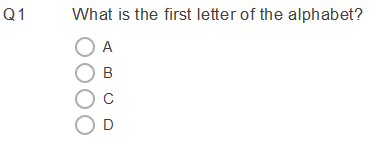### Step 2

To simplify the scoring process, the correct answer will always be the first answer to every question. We will use the Ordering option in the Variable Properties to Randomise the answers for people taking the quiz.

Right click on Q1 and select Variable Properties

Select Random for the type of Ordering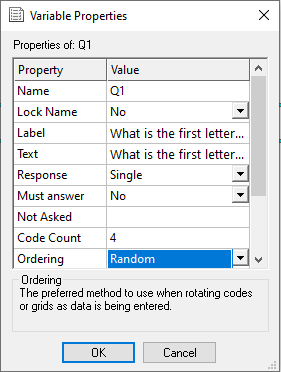### Step 3

Set up three more questions called Q2, Q3 and Q4, each with four possible answers (codes), the first answer being the correct one and randomise the order of the codes (repeat steps 1 and 2).

### Step 4

Click on the weights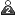button to open the Weights window.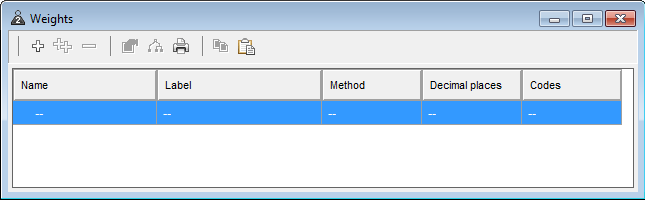Select the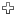button to create a new weight.
At the Weight details dialogue box, enter a Value of 1 for Code 1 and 0 for Code 2, 3 and 4. Name this as Score.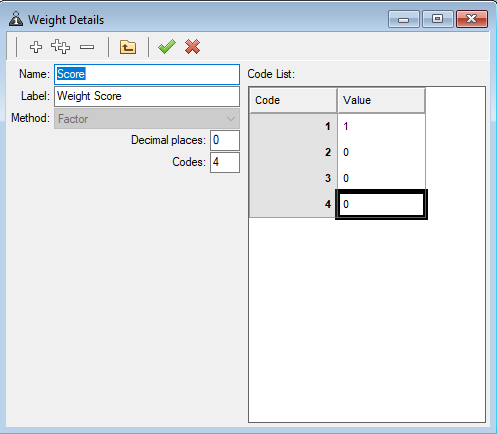Save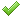the new weight and close the Weights dialogue.

### Step 5

Click View on the menu and choose Variables. Then clickto create a new derived variable which will be used to total the number of correct responses:

 Type: Derived Response Quantity Name: Total Label: Total Value for OK code: Score(Q1) + Score(Q2) + Score(Q3) + Score(Q4)

The value for the OK code assigns a score of 1 for a response to the first answer and 0 for a response to the second, third or fourth answers using the weight that we created in the previous step. This is then added together to give the score for all questions.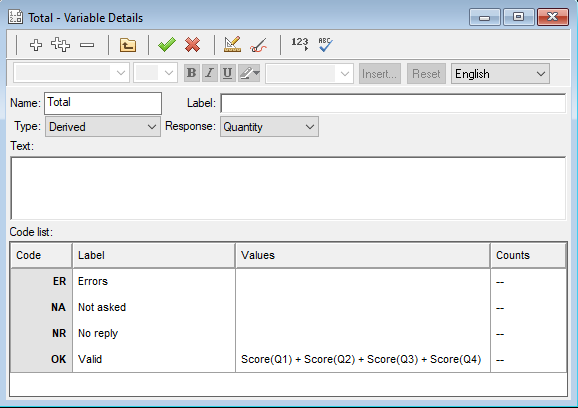Savethe new variable and close the Variables dialogue.

### Step 6

To display this total to the quiz taker, place the cursor in the text area of a question, title, or instruction, right click and choose Insert followed by Variable Field.

In the New variable field dialogue, select Total from the variable drop down and click OK.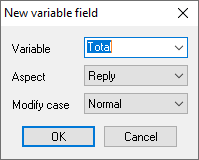This will appear like this in your design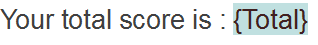When the quiz is completed, the total score will appear on a new page.The quiz is now ready and can be published and uploaded to Snap WebHost.

As a Snap user, you can download a 10 question quiz template to create your own quizzes.

Here are some examples of the quizzes we have created.

If there is a topic you would like a worksheet on, email to snapideas@snapsurveys.com